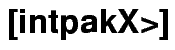Verified Numerics meets Computer Algebra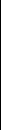intpakX combines Interval Arithmetic with the features of a Computer Algebra System. It offers a variety of algorithms for the use with Maple(TM). Thus, you can do verified computations and at the same time get your results graphically visualized.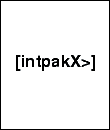Functional Range View a list of the most important functions and operators intpakX offers. View >>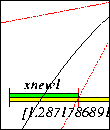Interval Newton Method The Interval version of the Newton Method lets you find all zeros of a continuously differentiable function and moreover gives you a proof for the existence of unique zeros. Example >>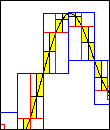2D Range Enclosure You can enclose the range of a real-valued function in one real variable using either equidistant or adaptive subdivision and either interval evaluation or the mean value form. Example >>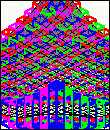3D Range Enclosure As well as for functions in one real variable, you can also compute range enclosures for real-valued functions in two variables and visualize the resulting intervals. Example >>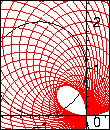Complex Disc Arithmetic Make use of complex disc arithmetic types and operations to enclose the range of a complex polynomial or compute the exponential function for disc intervals. Example >>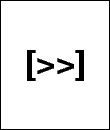Download - Literature - Contact Download intpakX or one of the available preprints or get in contact with us. go >>Wiss. Rechnen / Softwaretechnologie     FB Mathematik     Bergische Universität Wuppertal